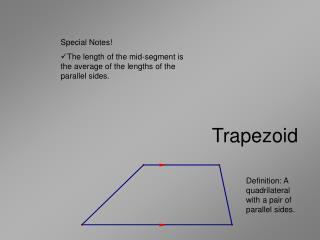DownloadDownload PresentationTrapezoid

# Trapezoid

Télécharger la présentation## Trapezoid

- - - - - - - - - - - - - - - - - - - - - - - - - - - E N D - - - - - - - - - - - - - - - - - - - - - - - - - - -
##### Presentation Transcript

1. Special Notes! • The length of the mid-segment is the average of the lengths of the parallel sides. Trapezoid Definition: A quadrilateral with a pair of parallel sides.

2. Definition: A quadrilateral opposites sides parallel. Parallelogram • Special Notes! • Adjacent angles are supplementary. • Opposite sides are congruent. • Opposite angles are congruent. • Diagonals bisect each other.

3. Isosceles Trapezoid Definition: A quadrilateral with two parallel sides (called bases) whose non parallel sides are congruent. • Special Notes! • Angles adjacent about the non-base sides are supplementary! • Length of mid-segment is the average of the bases.

4. Rhombus • Special Notes! • Opposite sides are parallel. • All of the properties of a parallelogram hold!  • Diagonals are perpendicular. Definition: A quadrilateral with four congruent sides.

5. Rectangle • Special Notes! • All the angles are right-angles. • All the properties of a parallelogram hold. Definition: A parallelogram with one right angle.

6. Special Notes! • Angles between congruent sides are called vertex angles. • Angle between non-congruent sides are called non-vertex angles. • Diagonals are perpendicular. • The diagonal between non-vertex angles is bisected by the diagonal between vertex angles. Kite NON-VERTEX ANGLES VERTEX ANGLES Definition: A quadrilateral with two pairs of congruent adjacent sides.

7. Definition: A rhombus that is a rectangle. Square • Special Notes! • Life's most perfect shape. • It a regular quadrilateral • All the properties of a parallelogram hold. • Diagonals are perpendicular. • A square is also a trapezoid. • A square is also a parallelogram. • A square is also a rhombus. • A square is also a rectangle. • A square is also a kite.